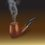# Find the Fallacy

Before starting reading this note please go through my note on uncountability of $R$. There I have shown that $R$, the set of real numbers, is uncountable. Now in this note, I will show a that there exists a bijection between $R$ and $N$. Well, obviously such bijection doesn't exists and my method has a fallacy, but I want you people to find out the fallacy yourself.

I will construct a function $f:N\rightarrow R$ as follows:

From $N$ choose an arbitrary element ${ n }_{ 1 }$ and map it to an arbitrary element ${ x }_{ 1 }$ from $R$.

Next choose an arbitrary element ${ n }_{ 2 }$ from $N-\{ { n }_{ 1 }\}$ and map it to arbitrary element ${ x }_{ 2 }$ of $R-\{ { x }_{ 1 }\}$ and continue the process.

Thus in the i-th step map the arbitrary element ${ n }_{ i }\in N-\{ { n }_{ 1 },{ n }_{ 2 },...,{ n }_{ i-1 }\}$ to an arbitrary element ${ x }_{ i }\in R-\{ { x }_{ 1 },{ x }_{ 2 },...,{ x }_{ i-1 }\}$.

Then, from the construction of $f$, $f$ is injective. Choose any two different elements from $N$, and observe that they are mapped to two elements of $R$ under $f$, which must be different (different because whenever in a step an element of $N$ is mapped to that of $R$, the latter is deleted from $R$ before proceeding to the next step). Again $f$ is surjective because choose any arbitrary element from $R$, see that it is the image of an element of $N$ (Since our process of construction of $f$ is infinite, this means at some step of our process we must have mapped an element of $N$ to that element of $R$).

So $f$ turns out to be bijective. Clearly, there is a strong fallacy in my considerations. Can anybody point it out?Note by Kuldeep Guha Mazumder
5 years ago

This discussion board is a place to discuss our Daily Challenges and the math and science related to those challenges. Explanations are more than just a solution — they should explain the steps and thinking strategies that you used to obtain the solution. Comments should further the discussion of math and science.

When posting on Brilliant:

• Use the emojis to react to an explanation, whether you're congratulating a job well done , or just really confused .
• Ask specific questions about the challenge or the steps in somebody's explanation. Well-posed questions can add a lot to the discussion, but posting "I don't understand!" doesn't help anyone.
• Try to contribute something new to the discussion, whether it is an extension, generalization or other idea related to the challenge.

MarkdownAppears as
*italics* or _italics_ italics
**bold** or __bold__ bold
- bulleted- list
• bulleted
• list
1. numbered2. list
1. numbered
2. list
Note: you must add a full line of space before and after lists for them to show up correctly
paragraph 1paragraph 2

paragraph 1

paragraph 2

[example link](https://brilliant.org)example link
> This is a quote
This is a quote
    # I indented these lines
# 4 spaces, and now they show
# up as a code block.

print "hello world"
# I indented these lines
# 4 spaces, and now they show
# up as a code block.

print "hello world"
MathAppears as
Remember to wrap math in $$ ... $$ or $ ... $ to ensure proper formatting.
2 \times 3 $2 \times 3$
2^{34} $2^{34}$
a_{i-1} $a_{i-1}$
\frac{2}{3} $\frac{2}{3}$
\sqrt{2} $\sqrt{2}$
\sum_{i=1}^3 $\sum_{i=1}^3$
\sin \theta $\sin \theta$
\boxed{123} $\boxed{123}$

Sort by:

Well, you need to ensure that$f$ is indeed a function. Where have you done that?

- 5 years ago

Every natural number is mapped to exactly one real number. That's enough to prove that it is a function.

- 5 years ago

Consider the same argument with the position of $\mathbb{N}$ and $\mathbb{R}$ reversed. Will you say that in this case also $f$ (if we retain the same notation) is a function?

- 5 years ago

Yes, of course.

EDIT: Actually, $f$ won't be a function, exactly because $|\mathbb{R}| > |\mathbb{N}|$. First, because $\mathbb{R}$ is uncountable, so the process won't finish even with infinite time. Second, since $|\mathbb{R}| > |\mathbb{N}|$, at some point there's no more element of $\mathbb{N}$ that hasn't been paired with.; the process of making $f$ is halted in this way. If we allow to pick any element of $\mathbb{N}$ and we can handle the "and continue arbitrarily" portion, then yes, $f$ becomes a function.

- 5 years ago

Yes, precisely that is the point.

- 5 years ago

Since our process of construction of $f$ is infinite, this means at some step of our process we must have mapped an element of $R$ to that element of $N$

This doesn't follow. Just consider the simple function $f : \mathbb{N} \to \mathbb{N}$ where $f(n) = n+1$. The process is infinite, yet 1 (or 0, if your natural numbers start with 0) has no pre-image.

- 5 years ago

Yes that's exactly the fallacy. The fallacy lies in the fact that the method by which the above function is being constructed doesn't guarantee surjectivity. Assume it to be surjective, then consider a closed subset of $R$ and apply the logic that I used while proving the uncountability of $R$.